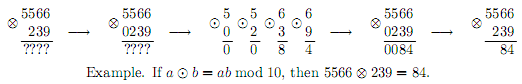Online JudgeProblem SetAuthorsOnline ContestsUser
Web Board
F.A.Qs
Statistical Charts
Problems
Submit Problem
Online Status
Prob.ID:
Register
Authors ranklist
Current Contest
Past Contests
Scheduled Contests
Award Contest
Register
Language:
Binary Operation
 Time Limit: 3000MS Memory Limit: 65536K Total Submissions: 239 Accepted: 67

Description

Consider a binary operationdefined on digits 0 to 9.: {0, 1, ..., 9} × {0, 1, ..., 9}{0, 1, ..., 9}, such that 00 = 0.
A binary operationis a generalization ofto the set of non-negative integers,:0+ ×0+0+. The result of ab is defined in the following way: if one of the numbers a and b has fewer digits than the other in decimal notation, then append leading zeroes to it, so that the numbers are of the same length;
then apply the operationdigit-wise to the corresponding digits of a and b.Let us defineto be left-associative, that is, abc is to be interpreted as (ab)c.
Given a binary operationand two non-negative integers a and b, calculate the value of a(a + 1)(a + 2)...(b - 1)b.

Input

The first ten lines of the input file contain the description of the binary operation. The i-th line of the input file contains a space-separated list of ten digits - the j-th digit in this list is equal to (i - 1)(j - 1).
The first digit in the first line is always 0.
The eleventh line of the input file contains two non-negative integers a and b (0 <= a <= b <= 1018).

Output

Output a single number – the value of a(a + 1)(a + 2)...(b - 1)b without extra leading zeroes.

Sample Input

0 1 2 3 4 5 6 7 8 9
1 2 3 4 5 6 7 8 9 0
2 3 4 5 6 7 8 9 0 1
3 4 5 6 7 8 9 0 1 2
4 5 6 7 8 9 0 1 2 3
5 6 7 8 9 0 1 2 3 4
6 7 8 9 0 1 2 3 4 5
7 8 9 0 1 2 3 4 5 6
8 9 0 1 2 3 4 5 6 7
9 0 1 2 3 4 5 6 7 8
0 10

Sample Output

15

Source

[Submit]   [Go Back]   [Status]   [Discuss]Home PageGo BackTo top